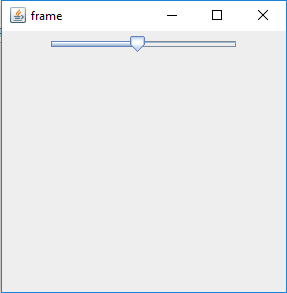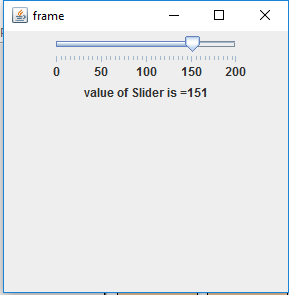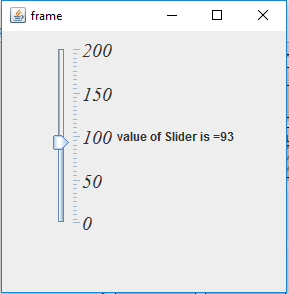# Java Swing | JSlider

JSlider is a part of Java Swing package . JSlider is an implementation of slider. The Component allows the user to select a value by sliding the knob withing the bounded value . The slider can show Major Tick marks and also the minor tick marks between two major tick marks, The knob can be positioned at only those points.

Constructor of the class are :

1. JSlider() : create a new slider with horizontal orientation and max and min value 100 and 0 respectively and the slider value is set to 50.
2. JSlider(BoundedRangeModel b) : creates a new Slider with horizontal orientation and a specified boundary range model.
3. JSlider(int o) : create a new slider with a specified orientation and max and min value 100 and 0 respectively and the slider value is set to 50.
4. JSlider(int min, int max) :create a new slider with horizontal orientation and max and min value specified and the slider value is set to the average of max and min value.
5. JSlider(int min, int max, int value) :create a new slider with horizontal orientation and max, min value and the slider Value specified .
6. JSlider(int o, int min, int max, int value) : create a new slider with orientation and max, min value and the slider Value specified .

Commonly used functions

1. setOrientation(int n) : sets the orientation of the slider to the specified value
2. setValue( int v) : sets the value of the slider to the specified value
3. setPaintTicks(boolean b) : the boolean value determines whether the ticks are painted on the slider or not
4. setPaintTrack(boolean b) : the boolean value determines whether the track are painted on the slider or not
5. setMajorTickSpacing(int n) : sets the spacing for major ticks .
6. setMinorTickSpacing(int n) : sets the spacing for minor ticks .
7. setFont(Font f) : set the font of the text for the slider
8. setMaximum(int m) : set the maximum value for the slider
9. setMinimum(int m) : sets the minimum value for the slider
10. updateUI() : Resets the UI property to a value from the current look and feel.
11. setValueIsAdjusting(boolean b) : Sets the model’s valueIsAdjusting property to boolean b.
12. setUI(SliderUI ui) : Sets the UI object which implements the Look and feel for this component.
13. setSnapToTicks(boolean b): if true is passed then the slider position is placed to nearest tick.
14. setModel(BoundedRangeModel n) : sets the BoundedRangeModel that handles the slider’s three fundamental properties: minimum, maximum, value.
15. setLabelTable(Dictionary l) : Used to specify what label will be drawn at any given value.
16. setInverted(boolean b): if passed true then the slider is set to inverted.
17. imageUpdate(Image img, int s, int x, int y, int w, int h): repaints the component when the image has changed.
18. setExtent(int extent) : sets the size of the range “covered” by the knob.
19. removeChangeListener(ChangeListener l): removes a ChangeListener from the slider.
20. getModel(): returns the BoundedRangeModel that handles the slider’s three fundamental properties: minimum, maximum, value.
21. getSnapToTicks() : returns true if the knob (and the data value it represents) resolve to the closest tick mark next to where the user positioned the knob.
22. getUI(): gets the UI object which implements the L&F for this component.
23. getPaintTrack() : returns if the tracks are painted or not.
24. getPaintTicks() : returns if ticks are painted or not
25. getPaintLabels() : returns whether labels are painted or not
26. getOrientation() : returns the orientation of the component.
27. getMinorTickSpacing() : returns the minor tick spacing
28. getMinimum(): returns the minimum value
29. getMaximum(): returns the maximum value
30. getMajorTickSpacing() : returns the major tick spacing.
31. addChangeListener(ChangeListener l) :dds a ChangeListener to the slider.\
32. createChangeListener() : create a change listener for the component
33. setUI(SliderUI ui) : sets the look and feel object that renders this component.
34. getUI() : returns the look and feel object that renders this component.
35. paramString() : returns a string representation of this JSlider.
36. getUIClassID() : returns the name of the Look and feel class that renders this component.
37. getAccessibleContext() : gets the AccessibleContext associated with this JSlider.

The Following programs will illustrate the use of JSlider

1. program to create a simple JSlider

 `// java Program to create a simple JSlider ` `import` `javax.swing.event.*; ` `import` `java.awt.*; ` `import` `javax.swing.*; ` `class` `solve ``extends` `JFrame { ` ` `  `    ``// frame ` `    ``static` `JFrame f; ` ` `  `    ``// slider ` `    ``static` `JSlider b; ` ` `  `    ``// main class ` `    ``public` `static` `void` `main(String[] args) ` `    ``{ ` `        ``// create a new frame ` `        ``f = ``new` `JFrame(``"frame"``); ` ` `  `        ``// create a object ` `        ``solve s = ``new` `solve(); ` ` `  `        ``// create a panel ` `        ``JPanel p = ``new` `JPanel(); ` ` `  `        ``// create a slider ` `        ``b = ``new` `JSlider(); ` ` `  `        ``// add slider to panel ` `        ``p.add(b); ` ` `  `        ``f.add(p); ` ` `  `        ``// set the size of frame ` `        ``f.setSize(``300``, ``300``); ` ` `  `        ``f.show(); ` `    ``} ` `} `

Output :2 . Program to create a slider with min and max value and major and minor ticks painted.

 `// java Program to create a slider with min and  ` `// max value and major and minor ticks painted. ` `import` `javax.swing.event.*; ` `import` `java.awt.*; ` `import` `javax.swing.*; ` `class` `solve ``extends` `JFrame ``implements` `ChangeListener { ` ` `  `    ``// frame ` `    ``static` `JFrame f; ` ` `  `    ``// slider ` `    ``static` `JSlider b; ` ` `  `    ``// label ` `    ``static` `JLabel l; ` ` `  `    ``// main class ` `    ``public` `static` `void` `main(String[] args) ` `    ``{ ` `        ``// create a new frame ` `        ``f = ``new` `JFrame(``"frame"``); ` ` `  `        ``// create a object ` `        ``solve s = ``new` `solve(); ` ` `  `        ``// create label ` `        ``l = ``new` `JLabel(); ` ` `  `        ``// create a panel ` `        ``JPanel p = ``new` `JPanel(); ` ` `  `        ``// create a slider ` `        ``b = ``new` `JSlider(``0``, ``200``, ``120``); ` ` `  `        ``// paint the ticks and tarcks ` `        ``b.setPaintTrack(``true``); ` `        ``b.setPaintTicks(``true``); ` `        ``b.setPaintLabels(``true``); ` ` `  `        ``// set spacing ` `        ``b.setMajorTickSpacing(``50``); ` `        ``b.setMinorTickSpacing(``5``); ` ` `  `        ``// setChangeListener ` `        ``b.addChangeListener(s); ` ` `  `        ``// add slider to panel ` `        ``p.add(b); ` `        ``p.add(l); ` ` `  `        ``f.add(p); ` ` `  `        ``// set the text of label ` `        ``l.setText(``"value of Slider is ="` `+ b.getValue()); ` ` `  `        ``// set the size of frame ` `        ``f.setSize(``300``, ``300``); ` ` `  `        ``f.show(); ` `    ``} ` ` `  `    ``// if JSlider value is changed ` `    ``public` `void` `stateChanged(ChangeEvent e) ` `    ``{ ` `        ``l.setText(``"value of Slider is ="` `+ b.getValue()); ` `    ``} ` `} `

Output :3 . Program to create a vertical slider with min and max value and major and minor ticks painted and set the font of the slider.

 `// java Program to create a vertical slider with  ` `// min and max value and major and minor ticks ` `// painted and set the font of the slider. ` `import` `javax.swing.event.*; ` `import` `java.awt.*; ` `import` `javax.swing.*; ` `class` `solve ``extends` `JFrame ``implements` `ChangeListener { ` ` `  `    ``// frame ` `    ``static` `JFrame f; ` ` `  `    ``// slider ` `    ``static` `JSlider b; ` ` `  `    ``// label ` `    ``static` `JLabel l; ` ` `  `    ``// main class ` `    ``public` `static` `void` `main(String[] args) ` `    ``{ ` `        ``// create a new frame ` `        ``f = ``new` `JFrame(``"frame"``); ` ` `  `        ``// create a object ` `        ``solve s = ``new` `solve(); ` ` `  `        ``// create label ` `        ``l = ``new` `JLabel(); ` ` `  `        ``// create a panel ` `        ``JPanel p = ``new` `JPanel(); ` ` `  `        ``// create a slider ` `        ``b = ``new` `JSlider(``0``, ``200``, ``120``); ` ` `  `        ``// paint the ticks and tarcks ` `        ``b.setPaintTrack(``true``); ` `        ``b.setPaintTicks(``true``); ` `        ``b.setPaintLabels(``true``); ` ` `  `        ``// set spacing ` `        ``b.setMajorTickSpacing(``50``); ` `        ``b.setMinorTickSpacing(``5``); ` ` `  `        ``// setChangeListener ` `        ``b.addChangeListener(s); ` ` `  `        ``// set orientation of slider ` `        ``b.setOrientation(SwingConstants.VERTICAL); ` ` `  `        ``// set Font for the slider ` `        ``b.setFont(``new` `Font(``"Serif"``, Font.ITALIC, ``20``)); ` ` `  `        ``// add slider to panel ` `        ``p.add(b); ` `        ``p.add(l); ` ` `  `        ``f.add(p); ` ` `  `        ``// set the text of label ` `        ``l.setText(``"value of Slider is ="` `+ b.getValue()); ` ` `  `        ``// set the size of frame ` `        ``f.setSize(``300``, ``300``); ` ` `  `        ``f.show(); ` `    ``} ` ` `  `    ``// if JSlider value is changed ` `    ``public` `void` `stateChanged(ChangeEvent e) ` `    ``{ ` `        ``l.setText(``"value of Slider is ="` `+ b.getValue()); ` `    ``} ` `} `

Output :Note : The above programs might not run in an online compiler please use an offline IDE

Attention reader! Don’t stop learning now. Get hold of all the important Java and Collections concepts with the Fundamentals of Java and Java Collections Course at a student-friendly price and become industry ready.

My Personal Notes arrow_drop_upCheck out this Author's contributed articles.

If you like GeeksforGeeks and would like to contribute, you can also write an article using contribute.geeksforgeeks.org or mail your article to contribute@geeksforgeeks.org. See your article appearing on the GeeksforGeeks main page and help other Geeks.

Please Improve this article if you find anything incorrect by clicking on the "Improve Article" button below.

Improved By : ManasChhabra2

Article Tags :
Practice Tags :

1

Please write to us at contribute@geeksforgeeks.org to report any issue with the above content.